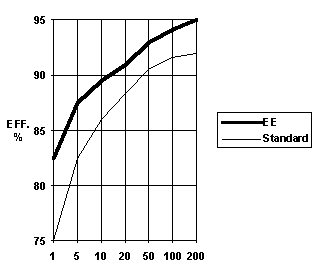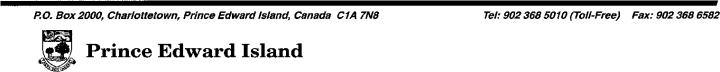November  1996                                                                                                                               Volume 2   Issue 19 Easy Comparison of Motor Cost/Payback Options     An important motor has just burned out, and you must replace it as soon as possible.  Do you have it rewound?  Do you replace it with a new motor?  Should that new motor have the same horsepower rating as the old one, or a higher or lower rating?  Should that new motor be an energy-efficient (EE) or standard-efficiency model? THE TECHNICAL ISSUES    To some extent, the choice will depend on technical matters.  Take horsepower rating, for example.  If you have already measured input current and voltage for each of your plant’s large motors (See Smart Energy User Issue 17) you will know whether this motor was overloaded or underloaded, and whether getting a smaller or larger replacement would make technical sense.  The buy-or-rewind choice has its technical side too.  The efficiency of a rewound motor is affected by the type of iron in its core and the temperature to which it was heated to loosen the old, burned-out windings.  Some motor rewinders are able to accurately control this temperature and keep it below the desired maximum of 650°F; others are not. THE COST/PAYBACK QUESTION     The cost/payback issue involves comparing the implementation cost and operating cost associated with the various ways of solving the problem:  1. Rewind the old motor.  2. Buy a standard replacement motor.  3. Buy an energy-efficient replacement motor.   Annual Operating Cost To analyze the operating cost of each option you need the following information: HP = rated full-load horsepower of the motor.         Motor Efficiency Comparison Standard and Energy-Efficient TypesMotor Horsepower               Eff= measured, rated, or estimated motor efficiency in per cent.  If possible, use the actual efficiency rating of the motor, as indicated on the motor nameplate or specified in the manufacturer’s published data.  Otherwise, estimate motor efficiency using the graph above.  The lower “Standard” curve shows the current minimum allowable efficiency for 3-phase 4-pole (~1800 rpm) motors under the Federal government’s Energy Efficiency Regulations.  The upper “EE” curve shows the minimum efficiency that a 4-pole motor must have to be called energy-efficient under the rules of the U.S. National Electrical Manufacturers’ Association (NEMA).  This curve has been proposed as a new Canadian minimum efficiency standard for all new 3-phase motors in this horsepower range.  If the proposal is adopted, “Standard” efficiency motors will no longer be available in Canada.  L = Load factor, or percent of full load at which the motor actually operates.  If you know Pin (the motor input power in kW under normal operating conditions) and Eff, then L can be calculated:      Pin x Eff L = ——————     0.746 x HP HRS = total hours per year that the motor runs at the load factor above. C = the unit cost of electricity per kWh — with demand charges, rebates, and taxes included.  To get this figure, take a recent electricity bill and divide the total dollar amount by the kWh total for that billing period.  The  formula for calculating annual operating cost is:                              L                  100 Annual Operating Cost = HP x —— x HRS x 0.746 x C x ——                            100                   Eff The annual operating cost for various repair/replacement options can be calculated using the above formula simply by plugging in the appropriate efficiency figure. Note: This simple formula gives satisfactory results if the motor’s load factor is fairly constant, and if the motor is in operation when the facility’s peak electrical demand occurs.  If these assumptions do not apply, then more complex calculations are necessary.  Call Mike Proud or Ron Estabrooks of the Energy and Minerals Section at 368-5010 (toll free) for a suggested procedure. Simple Payback     In general, simple payback is the length of time it takes annual operating cost savings to accumulate to an amount equal to the cost of implementing the energy conservation measure.  The equation is:                Implementation cost Payback = ————————————————            Annual Operating Cost Savings In the case of a burned-out motor, the payback calculation is used to compare two possible ways of dealing with the problem — one having higher implementation cost but greater annual savings than the other.  Here, because some course of action must be taken to solve the problem, we are interested in the time required to pay back the difference in implementation cost:              Difference in Implementation cost Payback = ——————————————————           Difference in Annual Operating Cost This formula can be used to compare any two of our three options: rewind the burned-out motor, replace it with a new standard-efficiency motor, or replace it with a new EE motor. AN EXAMPLE     Let’s assume that a 20 hp standard-efficiency motor just burned out.  We know that the motor operates 4000 hours per year (333 hours per month) at a load factor of 75%, and assume that the motor’s efficiency is the 88% indicated by the “Standard” curve.  The motor does contribute to peak demand, and C, the plant’s unit cost of electrical energy, is 11.2 cents per kWh.  We want to compare having the motor rewound with getting a new energy-efficient replacement motor.     A motor rewinding firm has quoted \$675 plus taxes (\$794 total) for the rewinding job.  Because the motor is only a few years old, and this firm will do the burnout at 650°F or less, we assume that motor efficiency after rewinding would still be 88%. The annual operating cost for this option is:                      75                       100 Annual Op. Cost = 20 x —— x 4000 x 0.746 x 0.112 x ——                      100                      88                = \$5697     The best price on an energy-efficient motor is \$1187 plus taxes (\$1397 total), and this particular motor has exceptionally good efficiency: 92.6% rather than the 91% indicated by the EE curve.  The annual cost of operating this motor would be:                       75                      100 Annual Op. Cost = 20 x —— x 4000 x 0.746 x 0.112 x ——                      100                      92.6                = \$5414     The difference in annual operating cost would be \$5697 minus \$5414, or \$283.   The difference in implementation cost would be \$1397 minus \$794, or \$603.  Thus:            \$603 Payback = ——— = 2.1 years           \$283 If you have questions about these calculations or about energy-efficient motors, call Mike Proud or Ron Estabrooks at 368-5010 (toll free)